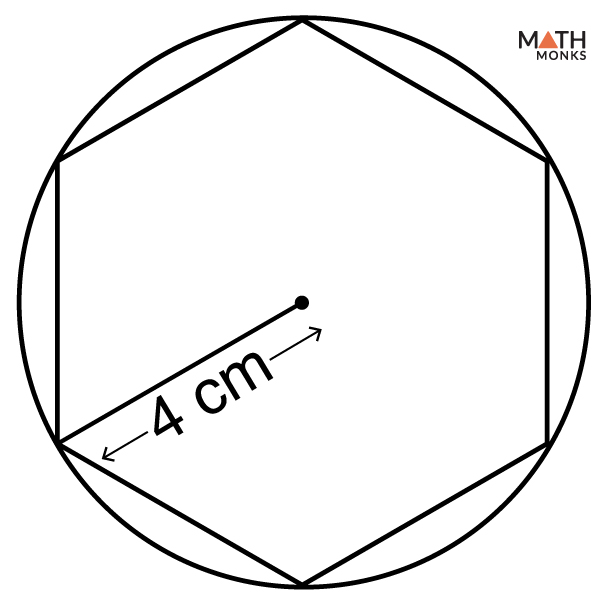# Hexagon Inscribed in a Circle

A hexagon inscribed in a circle is a hexagon so placed within the circle that its six vertices touch the circumference. In such a situation, the circle circumscribes or restricts the hexagon within its limit of circumference. A circle can only circumscribe a regular hexagon. Let us see the diagram of a regular hexagon inscribed in a circle.

## How to Inscribe a Hexagon in a Circle

Let us learn how to construct a regular hexagon inscribed in a circle. Given below is a link showing how to inscribe a hexagon in a circle.

## Solved Examples

### Finding Area

Finding the area of a hexagon inscribed in a circle when RADIUS of CIRCLE is knownIf a regular hexagon is inscribed in a circle with a radius of 4 cm, what is the area of the hexagon?

Solution:

As we know,
Side (s) of a hexagon inscribed in a circle = radius (r) of circumscribed circle
Thus, Area (A) = 3√3/2 × (r)2 , here r = 4 cm
= 3√3/2 × 4 × 4
= 24√3 sq. cm

### Finding Perimeter

Finding the perimeter of a hexagon inscribed in a circle when RADIUS of CIRCLE is known

Find the perimeter of a hexagon inscribed in a circle with radius 6 cm.

Solution:

As we know,
Side (s) of a hexagon inscribed in a circle = radius (r) of circumscribed circle
Perimeter (P) = 6r, here r = 6 cm
= 6 × 6
= 36 cm